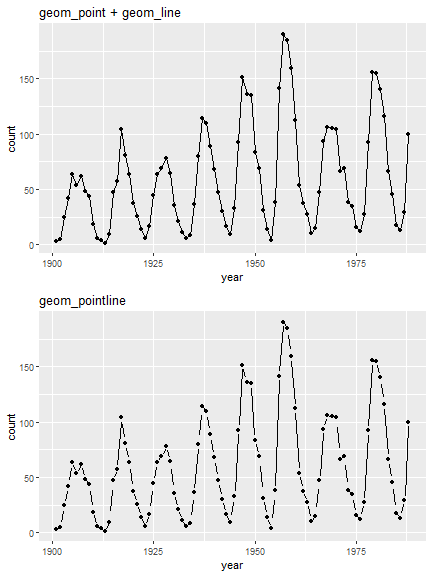In this vignette, we will demonstrate the two geoms: geom_pointline and geom_siderange.

geom_pointline simply combines geom_point and geom_line, while geom_pointpath combines geom_point and geom_path. The difference is that geom_line draws its lines through the data points, geom_pointline leaves a small aesthetic gap between the symbol and the line:

library(ggplot2)
library(lemon)
library(gridExtra)

data(sunspot.year)
sunspots <- data.frame(count=as.numeric(sunspot.year), year=seq.int(start(sunspot.year), end(sunspot.year)))
sunspots <- subset(sunspots, year > 1900)

point <-  ggplot(sunspots, aes(x=year, y=count)) + geom_point() + geom_line() + labs(title='geom_point + geom_line')
pointline <- ggplot(sunspots, aes(x=year, y=count)) + geom_pointline(distance=unit(3, 'pt'), threshold=0.2) + labs(title='geom_pointline')
grid.arrange(point, pointline)